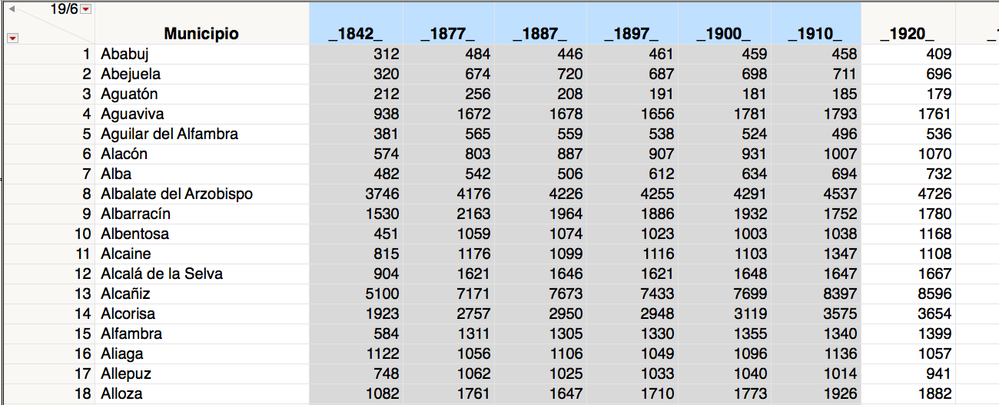Choose Language Hide Translation Bar
Highlighted

## In a loop how to indicate other column that [I]

Hi.
Again I have to ask for help. I have written a script that calculates the percentage of change between the values ​​of a numeric column and the next one. Unfortunately I get an error: "Column 1897_Canvi Formula Interrupted
Cannot convert argument to a number [or matrix] 1 times At rows: {1} Operation: Subtract, numericColNames [X] - numericColNames [i]
Formula evaluation errors have been ignored "

This are the numericColNamesn:
{"_1842 _", "_ 1877_", "_1887_", "_1897_", "_1900_", "_1910_", "_1920_", "_1930_", "_1940 _", "_ 1950_", "_1960_", "_1970_", " _1981_ "," _1991_ "," _2001_ "," _2011_ "," _2018_ "}

This is the script in question:

``````Names Default To Here( 1 );
dt = Current Data Table();
numericColNames = dt << get column names( string, numeric );
For( i = 2, i <= N Items( numericColNames ), i++,
Eval(
Substitute(
Expr(
New Column( Char( numericColNames[i] ) || "_Canvi",
Numeric,
Continuous,
Format( "Percent", 12, 1 ),
X = i - 1,
Formula( (numericColNames[X] - numericColNames[i]) / numericColNames[X] )
)
),
Expr( __col__ ), Parse( ":Name (\!" " || numericColNames[i] || "\!")" )
)
)
);``````
4 REPLIES 4
Highlighted

## Re: In a loop how to indicate other column that [I]

You are not setting up the Substitute() function correctly.

Substitute and Substitute Into
The Substitute() function returns a copy of a string with a replacement expression. The
Substitute Into() function changes the original string with a replacement expression.
For example, consider the following script:

`str1 = str2 = "All things considered";str3 = Substitute( str1, "All", "Some" );// str3 holds the result of the Substitute, and str1 is not changedstr4 = Substitute Into( str2, "All", "Some" );// Substitute Into returns nothing, so str4 is missing, and str2 is changed// and now holds the result of Substitute IntoShow( str1, str2, str3, str4 );`

This script returns the following output:
str1 = "All things considered";
str2 = "Some things considered";
str3 = "Some things considered";
str4 = .;
I have reworked you script, to what I am guessing will be what you want

``````Names Default To Here( 1 );
dt = Current Data Table();
numericColNames = dt << get column names( string, numeric );
For( i = 2, i <= N Items( numericColNames ), i++,
Eval(
Substitute(
Expr(
New Column( Char( numericColNames[i] ) || "_Canvi",
Numeric,
Continuous,
Format( "Percent", 12, 1 ),
X = i - 1,
Formula( (__colX__ - __coli__ / __colX__ )
)
),
Expr( __coli__ ), Parse( ":Name (\!" " || numericColNames[i] || "\!")" ),
Expr( __colX__ ), Parse( ":Name (\!" " || numericColNames[X] || "\!")" )
)
)
);``````
` `

The Substitute() function documentation was copied from the Scripting Guide

Help==>Books==>Scripting Guide

Jim
Highlighted

## Re: In a loop how to indicate other column that [I]

First of all, thanks for your help. When I run your script I get the error:

In the following script, error marked by / * ### * /
Substitute / * ### * / (Expr (...

This part of the script was you suggested in a past post since the name of the columns were whole numbers like 1980. The substitute could be eliminated if instead of 1980 it was "_1980_".

What do the operators "\!" after ": Name".

Highlighted

## Re: In a loop how to indicate other column that [I]

The 1980 column name causes an issue which is fixed by putting a :Name("1980") around the column specification.  It is not why the Substitute() function is used.  The Substitute() function is there, because you do not want the formula that is saved for the column to have the value

``(numericColNames[X] - numericColNames[i]) / numericColNames[X] )``

but rather to have the value

``( __1980__ - __1960__ / __1980__ )``

If the formula has the first syntax I specified, it will mean that the formula will fail as soon as your JMP session changes any of the values for: i, X, numericColNames.  In other words, if you save your data table, end your JMP session, and then startup JMP again, none of the variables i, X, or numericColNames will be in your new JMP session, so that when you open the data table, all of the columns that have formulas that reference those variables will fail.

Finally, I am not sure what is the issue you are currently finding.  If you could provide a sample of your data, I will at least have a chance of figuring out the issue.

Jim
Highlighted

## Re: In a loop how to indicate other column that [I]

Hello again. What I intend is to calculate the percentage change in the number of inhabitants in the successive censuses that have been carried out in the municipalities of the province of Teruel (Spain) over time. My intention is to compare a census with the following census. In the first column of the data table that I enclose (partial) the names of the municipalities are recorded, in the following six the inhabitants of each municipality appear in the different censuses. To calculate the exchange rate, I calculate the difference between one census and the next, the result is divided by the first census and then I express the value obtained as a percentage. i.e. (_1842_ - _1877_) / _1842_Article Labels

There are no labels assigned to this post.